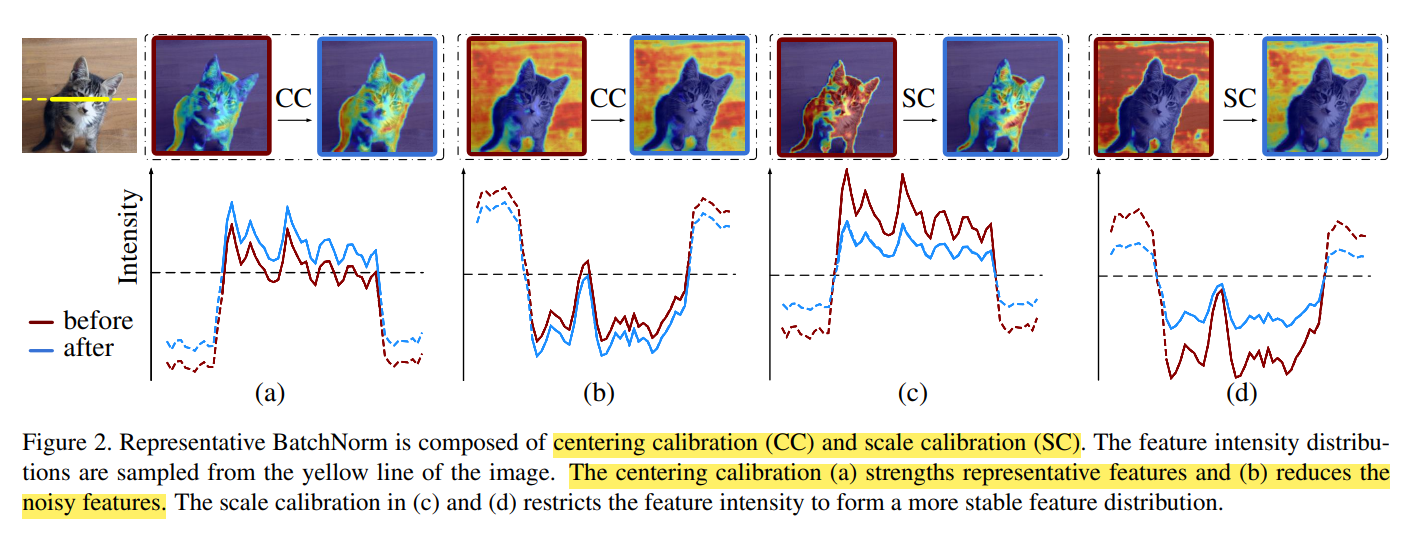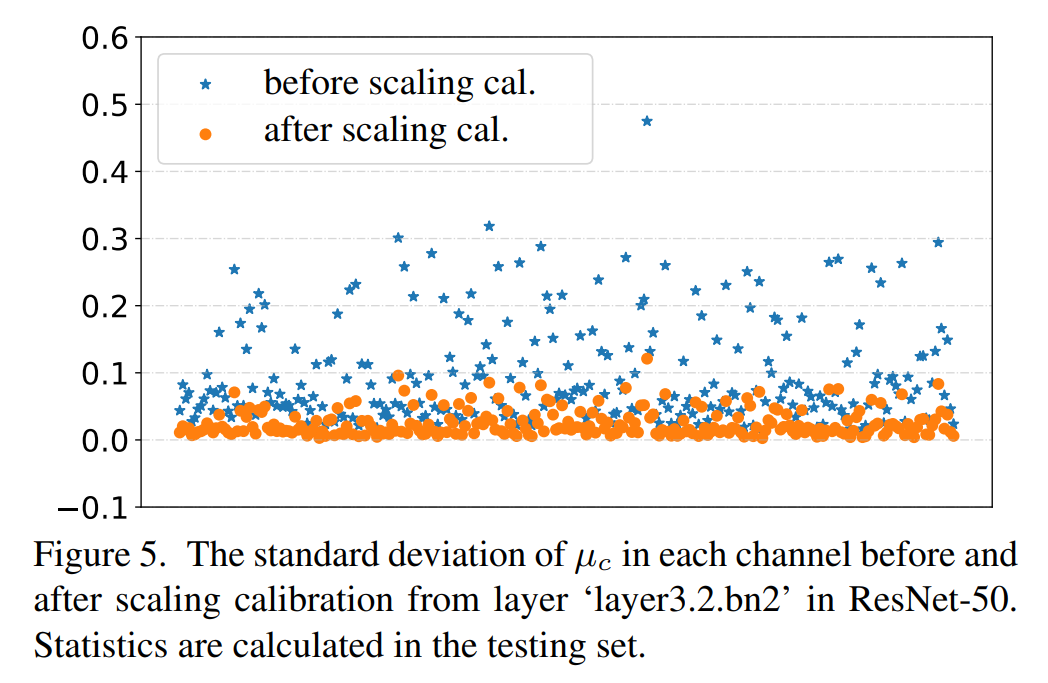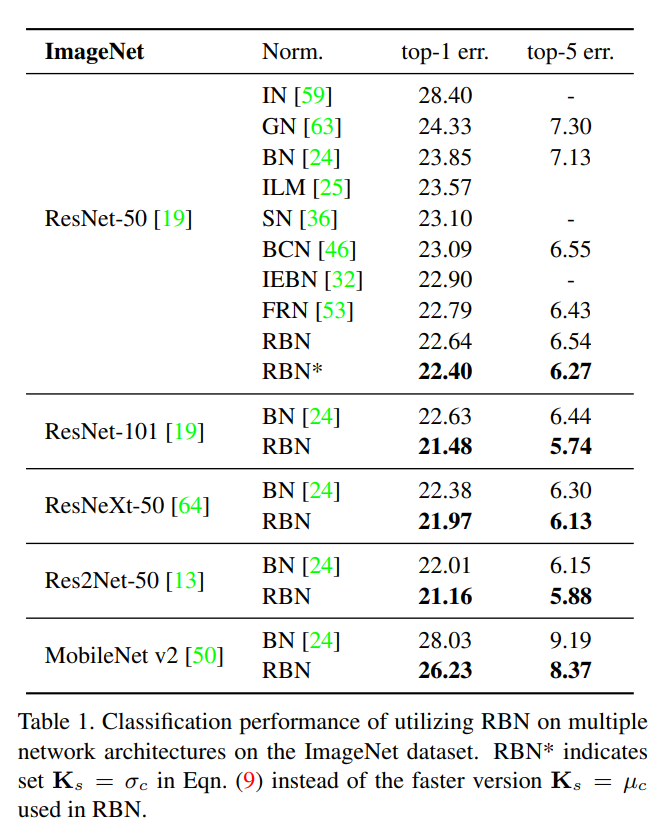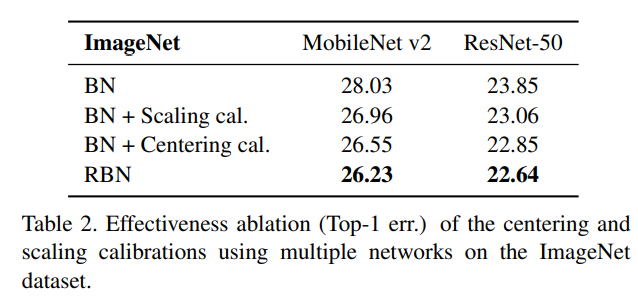# 引言¶

BatchNorm模块能让模型训练更加稳定，因而被广泛使用。它的中心化以及缩放步骤需要依赖样本统计得到的均值和方差，而这也导致了在归一化的过程，忽视了各个实例的区别。其中，中心化步骤是为了增强信息特征，减少噪声。而缩放步骤是为了让特征服从一个稳定的分布。考虑到不同实例有不同特点，我们引入了简单有效的特征校准步骤（feature calibration scheme），改进得到Representative BatchNorm，在各大图像任务均有一定的提升。

# BN的缺点¶

BatchNorm公式如下，它将特征缩放为一个均值为0，方差为1的分布

BN(x) = \gamma*\frac{(x-mean)}{\sqrt{var}}+\beta

BatchNorm的一个前提是，我们假定了不同实例对应的特征都服从相同的分布。 但实际中，存在以下两种情况不满足上述的假设：

1. 一个mini-batch里的统计信息（均值，方差）与总的训练集/测试集的统计信息不一致
2. 测试集中的数据实例不符合训练集的分布

• 当测试集的均值小于running-mean，BN会错误地移除掉具有代表性的特征
• 当测试集的均值大于running-mean，BN会“漏掉”特征中的噪声
• 当测试集的方差小于running-var，BN会导致特征的intensity过小
• 当测试集的方差大于running-var，BN会导致特征的intensity过大

# Representative Batch Normalization¶## Centering Calibration¶

X_{cm(n,c,h,w)} = X_{(n,c,h,w)} + w_m \odot K_m

### 公式推导¶

K_m = \frac{1}{HW} \sum_{h=1}^H{\sum_{w=1}^W} X_{(n,c,h,w)}

E(X) = E(K_{m})

E(X_{cm} ) =(1+W_m)*E(X)

X_{cal} = X - E(X_{cm}) 即输入减去中心校准过的均值 \\ X_{no} = X - E(X) 即输入减去均值

X_{cal} - X_{no} = X + w_m*K_m - (1+w_m)*E(X) - (X - E(X)) \\ = w_m(K_m - E(X))

• $w_m$ 大于0，且$K_m$ > $E(X)$，此时Representative Feature得到增强，反之亦然
• $w_m$ 小于0，且$K_m$ > $E(X)$，此时特征噪声会抑制，反之亦然

## Scaling Calibration¶

X_{cs(n,c,h,w)} = X_{(n,c,h,w)} *R(w_v \odot K_s + w_b)

### 公式推导¶

0 < R() < 1

Var(X_{cs}) < Var(X_{cs}*\tau) = \tau^2*Var(X_{cs})## 整体流程¶

X_{cm} = X + w_m \odot K_m

X_m = X_{cm} - E(X_{cm}) \\ X_s = \frac{X_m}{\sqrt{Var(X_{cm}) + \epsilon}}

X_{cs} = X_s*R(w_v \odot K_s + w_b)

Y = \gamma*X_{cs} + \beta

# 实验对比¶# 代码¶

import torch.nn as nn
import math
import torch
import numpy as np
import torch.nn.functional as F
class RepresentativeBatchNorm2d(nn.BatchNorm2d):
def __init__(self, num_features, eps=1e-5, momentum=0.1, affine=True,
track_running_stats=True):
super(RepresentativeBatchNorm2d, self).__init__(
num_features, eps, momentum, affine, track_running_stats)
self.num_features = num_features
### weights for affine transformation in BatchNorm ###
if self.affine:
self.weight = nn.Parameter(torch.Tensor(1, num_features, 1, 1))
self.bias = nn.Parameter(torch.Tensor(1, num_features, 1, 1))
self.weight.data.fill_(1)
self.bias.data.fill_(0)
else:
self.register_parameter('weight', None)
self.register_parameter('bias', None)

### weights for centering calibration ###
self.center_weight = nn.Parameter(torch.Tensor(1, num_features, 1, 1))
self.center_weight.data.fill_(0)
### weights for scaling calibration ###
self.scale_weight = nn.Parameter(torch.Tensor(1, num_features, 1, 1))
self.scale_bias = nn.Parameter(torch.Tensor(1, num_features, 1, 1))
self.scale_weight.data.fill_(0)
self.scale_bias.data.fill_(1)
### calculate statistics ###

def forward(self, input):
self._check_input_dim(input)

####### centering calibration begin #######
input += self.center_weight.view(1,self.num_features,1,1)*self.stas(input)
####### centering calibration end #######

####### BatchNorm begin #######
if self.momentum is None:
exponential_average_factor = 0.0
else:
exponential_average_factor = self.momentum

if self.training and self.track_running_stats:
if self.num_batches_tracked is not None:
self.num_batches_tracked = self.num_batches_tracked + 1
if self.momentum is None:  # use cumulative moving average
exponential_average_factor = 1.0 / float(self.num_batches_tracked)
else:
exponential_average_factor = self.momentum
output = F.batch_norm(
input, self.running_mean, self.running_var, None, None,
self.training or not self.track_running_stats,
exponential_average_factor, self.eps)
####### BatchNorm end #######

####### scaling calibration begin #######
scale_factor = torch.sigmoid(self.scale_weight*self.stas(output)+self.scale_bias)
####### scaling calibration end #######
if self.affine:
return self.weight*scale_factor*output + self.bias
else:
return scale_factor*output


### weights for centering calibration ###
self.center_weight = nn.Parameter(torch.Tensor(1, num_features, 1, 1))
self.center_weight.data.fill_(0)
### weights for scaling calibration ###
self.scale_weight = nn.Parameter(torch.Tensor(1, num_features, 1, 1))
self.scale_bias = nn.Parameter(torch.Tensor(1, num_features, 1, 1))
self.scale_weight.data.fill_(0)
self.scale_bias.data.fill_(1)


### calculate statistics ###


####### centering calibration begin #######
input += self.center_weight.view(1,self.num_features,1,1)*self.stas(input)
####### centering calibration end #######


...
output = F.batch_norm(
input, self.running_mean, self.running_var, None, None,
self.training or not self.track_running_stats,
exponential_average_factor, self.eps)


####### scaling calibration begin #######
scale_factor = torch.sigmoid(self.scale_weight*self.stas(output)+self.scale_bias)
####### scaling calibration end #######


if self.affine:
return self.weight*scale_factor*output + self.bias
else:
return scale_factor*output


# 总结¶